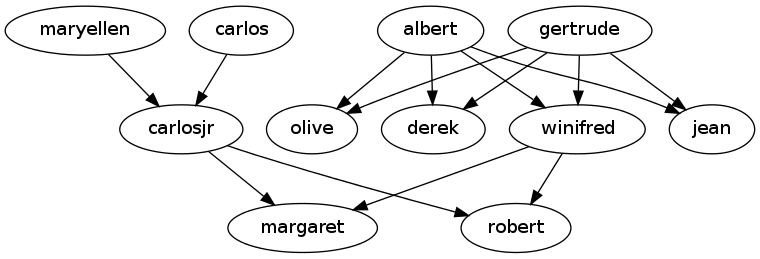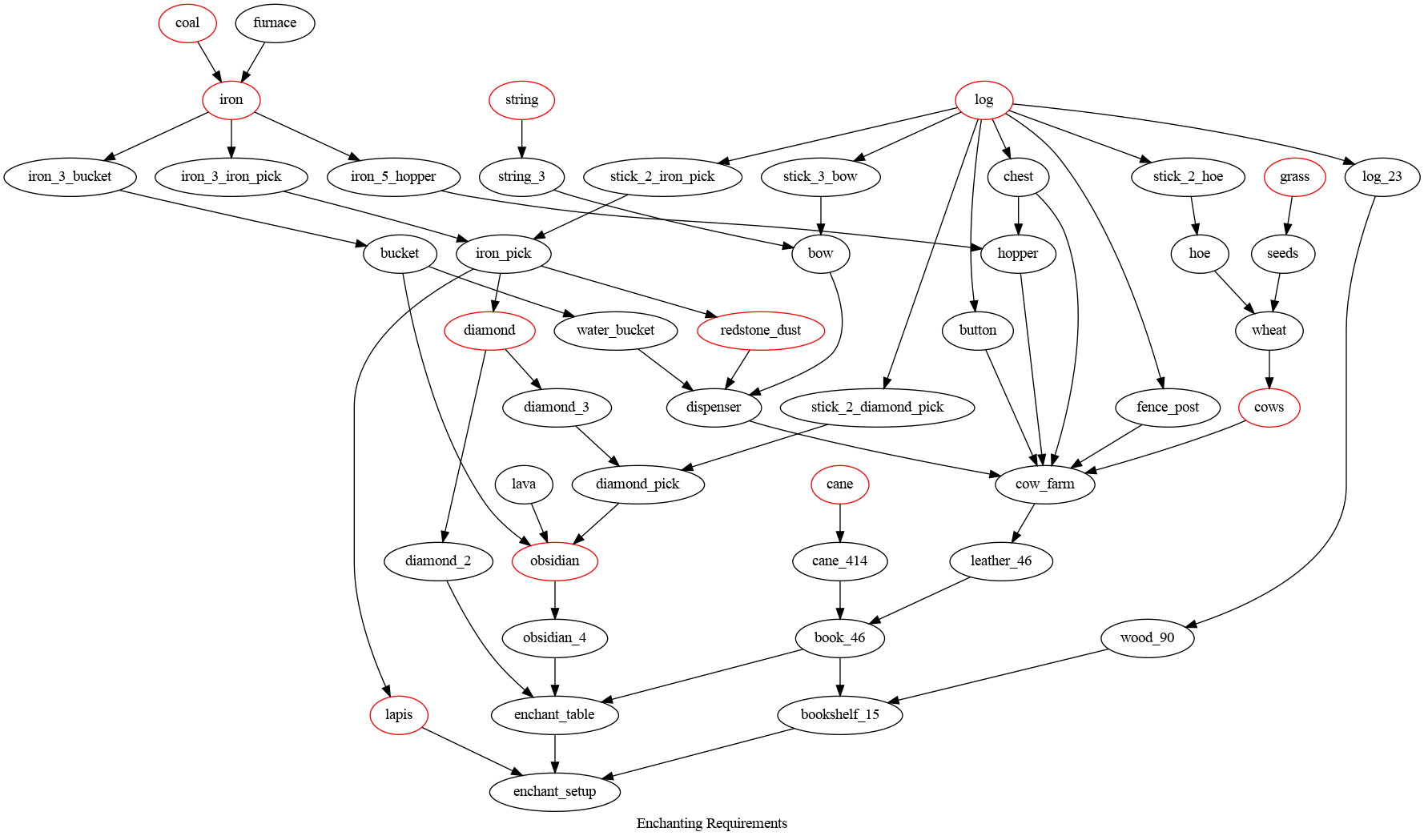Path Planning

• Another approach involves hubs (one is called "Hub Labels") and uses precomputed tables to speed things up. This is useful for road route planning. Here is a paper on the topic. This may be related to contraction hierarchies. Contraction hierarchies seem to be about caching shortcut calculations.

• A good resource from Stanford including sample implementations of A* and good explanations.

Graphviz

• -Tps

• -Tpng

• -Tsvg

Digraph

strict is if only one edge between nodes is allowed.

familytree.dot
// Sample Family Tree
strict digraph {
{maryellen;carlos} -> carlosjr
{winifred;carlosjr} -> {margaret;robert}
{albert;gertrude} -> {winifred;olive;derek;jean}
}

Process with this command.

dot -Tpng familytree.dot -o familytree.png

Which should produce something like this.Here’s another example.

digraph { label="Enchanting Requirements";
string[color="red"];
diamond[color="red"];
cane[color="red"];
iron[color="red"];
obsidian[color="red"];
cows[color="red"];
log[color="red"];
redstone_dust[color="red"];
lapis[color="red"];
grass[color="red"];
coal[color="red"];
furnace->iron;
coal->iron;
iron_pick->lapis;
iron->iron_3_bucket;
iron->iron_3_iron_pick;
log->stick_2_iron_pick;
stick_2_iron_pick->iron_pick;
iron_3_iron_pick->iron_pick;
string->string_3;
string_3->bow;
log->stick_3_bow;
stick_3_bow->bow;
iron_3_bucket->bucket;
bucket->water_bucket;
iron_pick->redstone_dust;
redstone_dust->dispenser;
bow->dispenser;
water_bucket->dispenser;
dispenser->cow_farm;
log->fence_post;
log->button;
grass->seeds;
seeds->wheat;
log->stick_2_hoe;
stick_2_hoe->hoe;
hoe->wheat;
iron->iron_5_hopper;
iron_5_hopper->hopper;
chest->hopper;
log->chest;
chest->cow_farm;
fence_post->cow_farm;
button->cow_farm;
wheat->cows;
cows->cow_farm;
hopper->cow_farm;
iron_pick->diamond;
diamond->diamond_2;
diamond->diamond_3;
diamond_2->enchant_table;
log->stick_2_diamond_pick;
stick_2_diamond_pick->diamond_pick;
diamond_3->diamond_pick;
cow_farm->leather_46;
leather_46->book_46;
cane->cane_414;
cane_414->book_46;
log->log_23;
log_23->wood_90;
wood_90->bookshelf_15
book_46->bookshelf_15
lava->obsidian;
bucket->obsidian;
diamond_pick->obsidian;
obsidian->obsidian_4;
obsidian_4->enchant_table;
book_46->enchant_table;
lapis->enchant_setup;
enchant_table->enchant_setup;
bookshelf_15->enchant_setup;
}RT Graph 3D

If Graphviz isn’t quite right because your graphs are really very 3 dimensional, "RTgraph3D will let you create dynamic graphs in 3D." One potential application of this is molecules. I haven’t tried it out myself but it’s worth noting.

Jargon

There sure are a lot of terms of art that need very careful definition. Here are many of them in one place.

graph

Set of edges and a set of vertices and a function that relates them.

simple graph

No loop backs or multiple edges. Also called "multigraph". Generally finite number of edges and vertices.

cycle graph

n edges and n vertices. Imagine a circle approximated by points along it (though there are other uglier ways to plot it).

When there is an edge connecting a pair.

Have at least one vertex in common.

incident

e is incident to v and w if v and w are connected by e. Also v and w are incident to e in that case.

degree of vertex

Number of edges which are incident to it. A loop counts as 2. A.k.a. "valency" in chemical structures (carbon=4, hydrogen=1).

n-regular

A graph where every vertex has the same degree, n.

null graph

Edge set is empty.

complete graph

Every pair of distinct vertices is joined by an edge. Represented as Kn where there are n edges connecting all of n vertices to each other.

bipartite graph

The vertices are divided into 2 sets and every edge goes from one set to the other.

complete bipartite graph

A bipartite graph where every vertex of set 1 is connected to every vertex of set 2.

A computer-friendly representation of a graph as a n`x`n matrix such that a~ij~ is the number of edges connecting vertices i and j. It’s possible that this could alternatively mean a matrix populated with a 1 or 0 depending on if there is a connection. This is true by default in a "complete graph".

isolated vertex

No edges connect to it.

connected

If any vertex can reach any other. No islands basically.

path

An edge traversal sequence where all traversed edges are distinct. Vertices can be revisited. Not all vertices or edges of a graph need participate for there to be a path.

simple path

A path where the vertices (with the possible exception of the first and last) are also distinct, i.e. visited only once.

closed path

V0 = Vn , i.e. the beginning vertex and end vertex is the same.

cycle

A.k.a. curcuit. A closed simple path containing at least one edge.

Eulerian path

A closed path which includes every edge.

Eulerian graph

A graph which contains at least one Eulerian path. A connected graph is Eulerian if and only if every vertex has an even degree. Hence there is no solution to Koenigsberg Bridge quandary.

Hamiltonian cycle

A cycle which passes through every vertex. Complete necessary and sufficient conditions for a graph to be Hamiltonian are an unsolved problem. However, a sufficient condition is if the number of vertices, n, is >= 3 and the degree is >= .5*n, then the graph is Hamiltonian. A graph can still be Hamiltonian without satisfying this theorem.

isomorphism

When two graphs share the same essential structure even if that structure is enumerated differently. Are all the things possible (routes) in one graph possible in the other? Isomorphic. To demonstrate isomorphic relationship, an isomorphism must be found (necessary and sufficient). To show they are not isomorphic, any of the graph theory properties (number of vertices/edges, degree, simple, Eulerian, Hamiltonian) shown to not match is sufficient. In chemistry a structural isomer is a different graph of the same edges (atoms); these are not isomorphic.

tree

A connected graph containing no cycles.

spanning tree

In a graph with vertex set V, a subgraph which is a tree and also has a vertex set V (all of them, no cycles).

minimum spanning tree

A spanning tree which is the smallest possible (total edge lengths).

Steiner tree

Similar to a minimum spanning tree but with the possibility of adding additional nodes not part of the original set of nodes.

full binary tree

One vertex has degree 2 (top or root node) and all other vertices have degree 3 (branch or decision nodes) or 1 (leaf nodes). There are always 2 or more leaf nodes than decision nodes.

level

Level of a vertex in a tree is the length of the path jointing that vertex and the root vertex. The level of the tree is the maximum level of all vertices in a tree.

bridge

Edge that will divide a graph into two graphs if removed.